Ex 6.5

Chapter 6 Class 10 Triangles
Serial order wise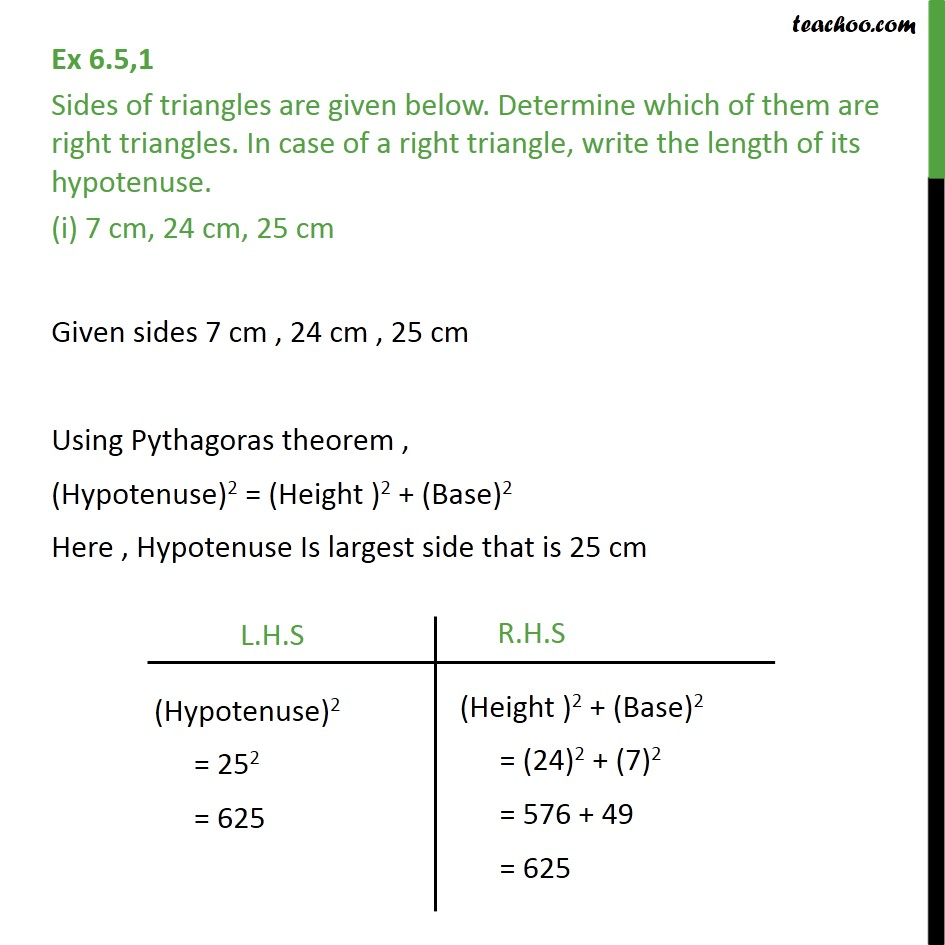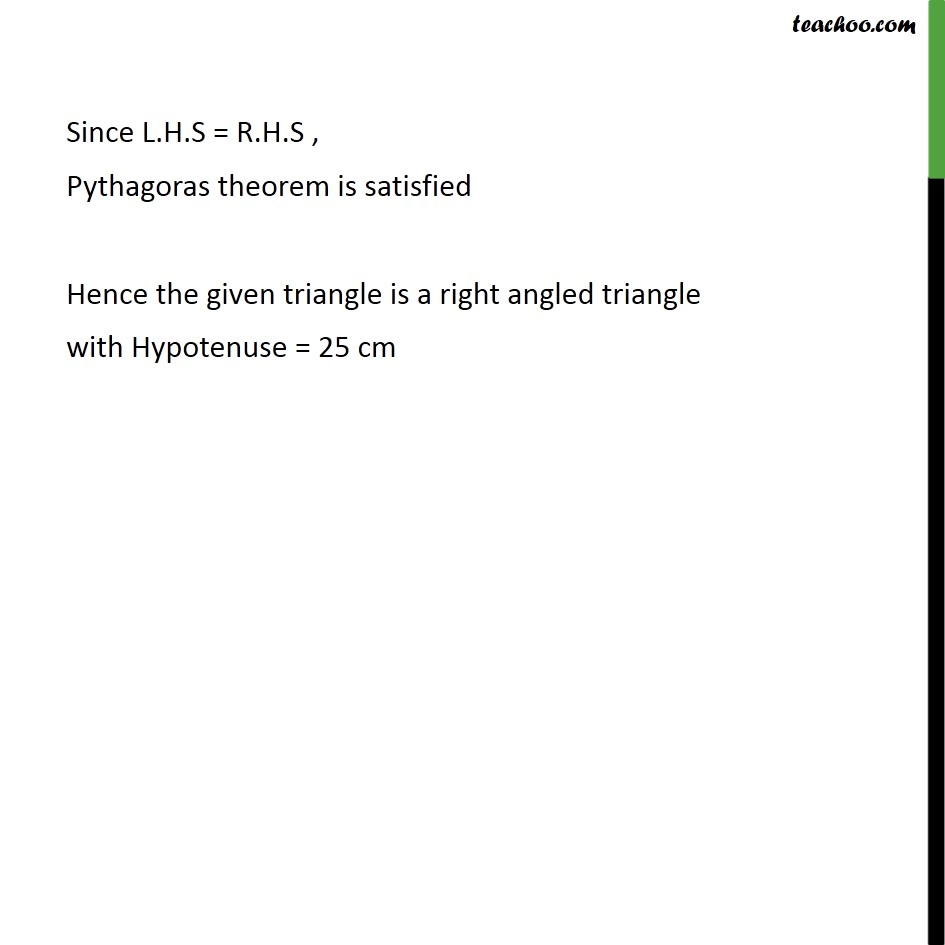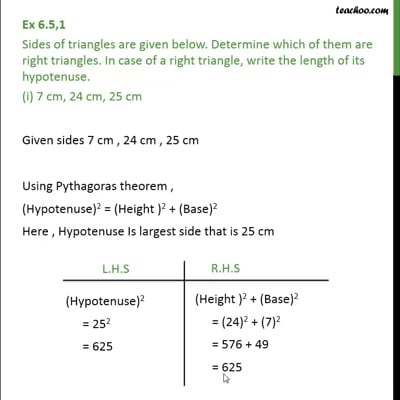This video is only available for Teachoo black users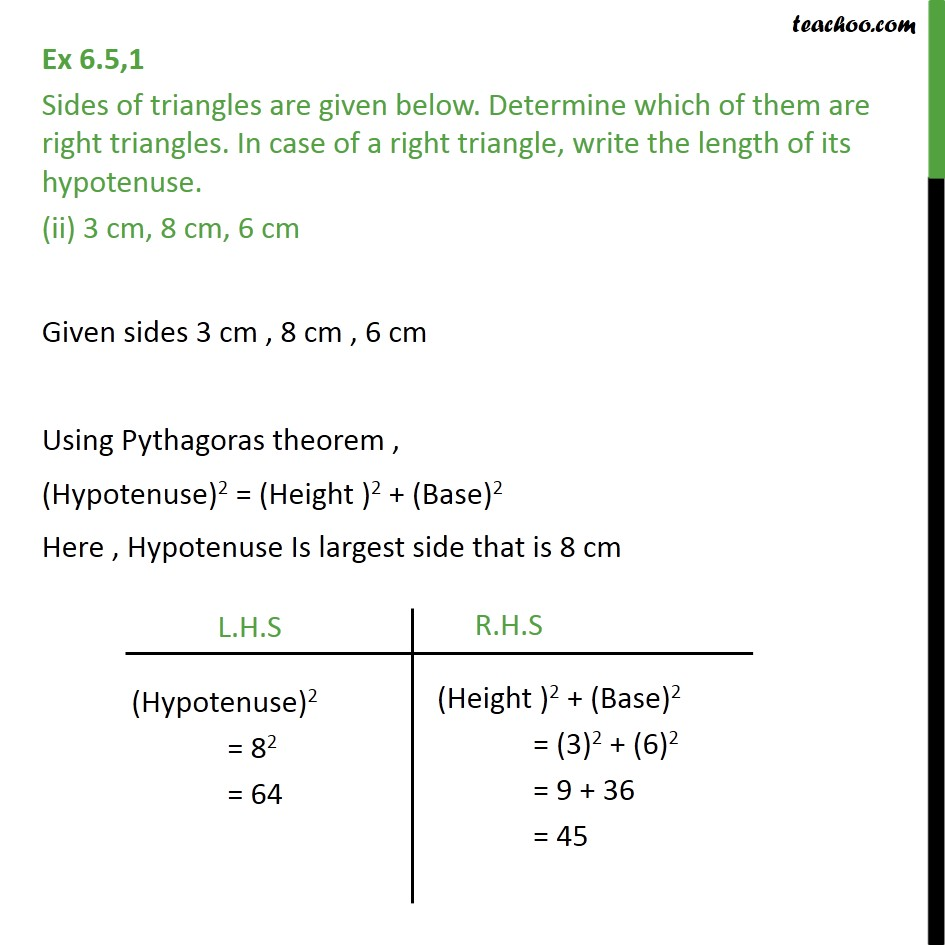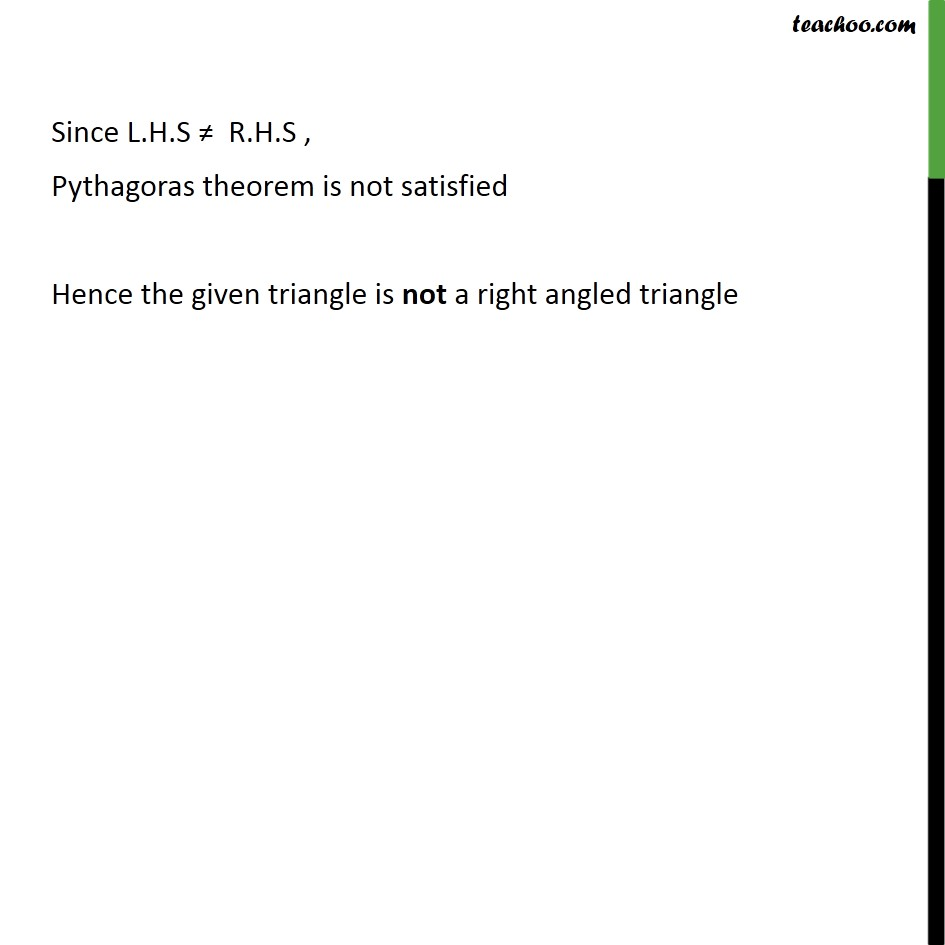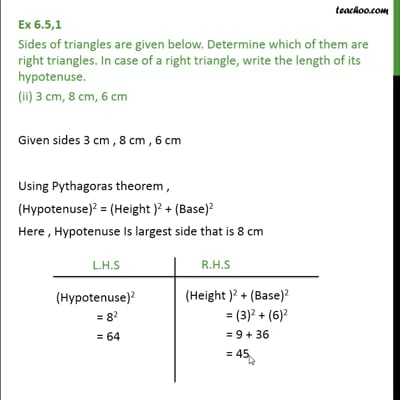This video is only available for Teachoo black users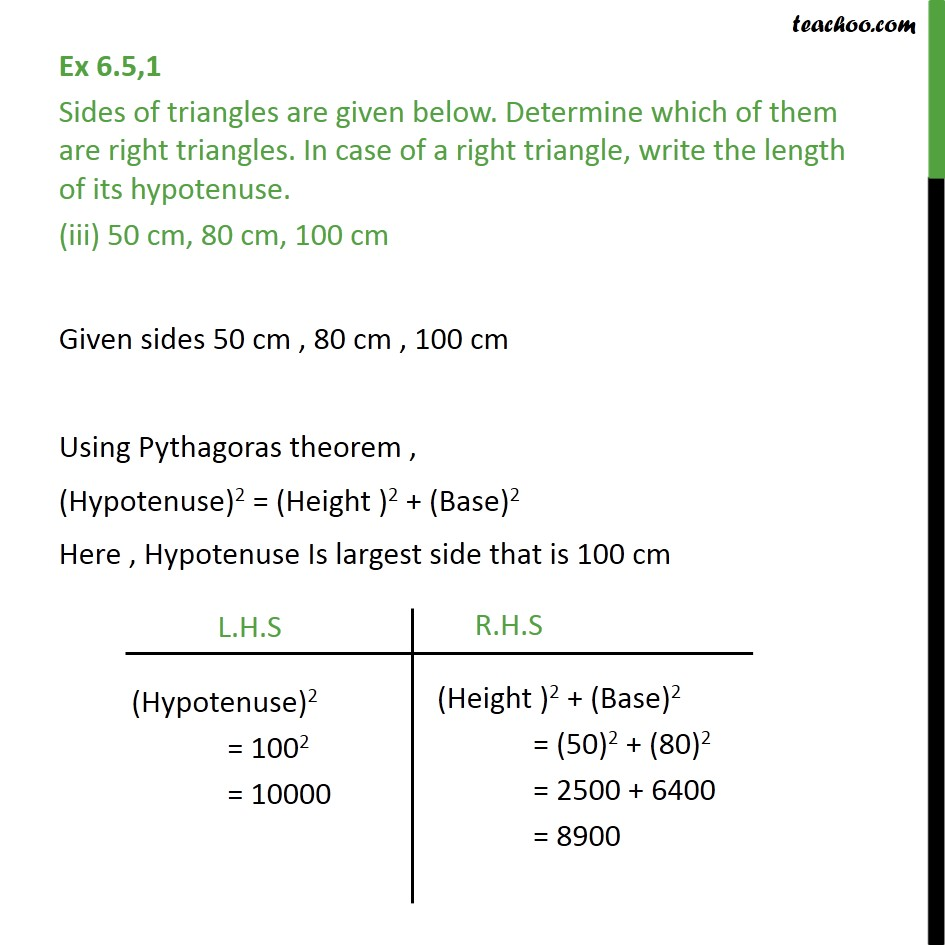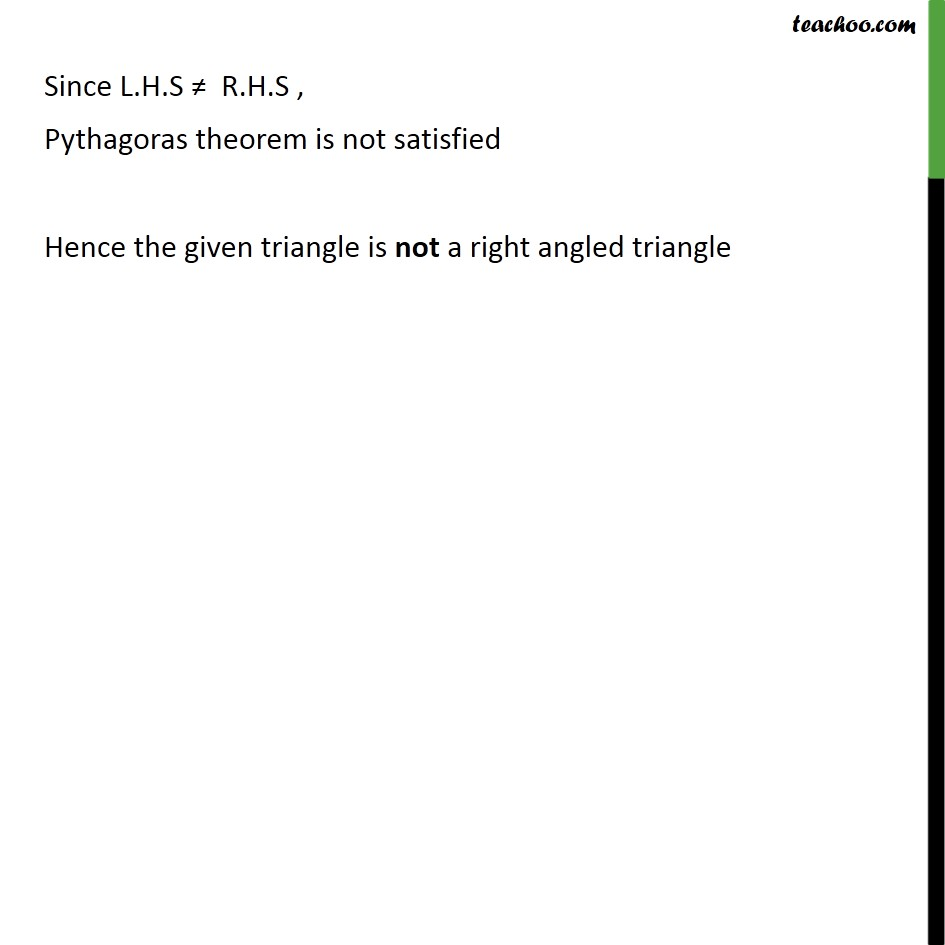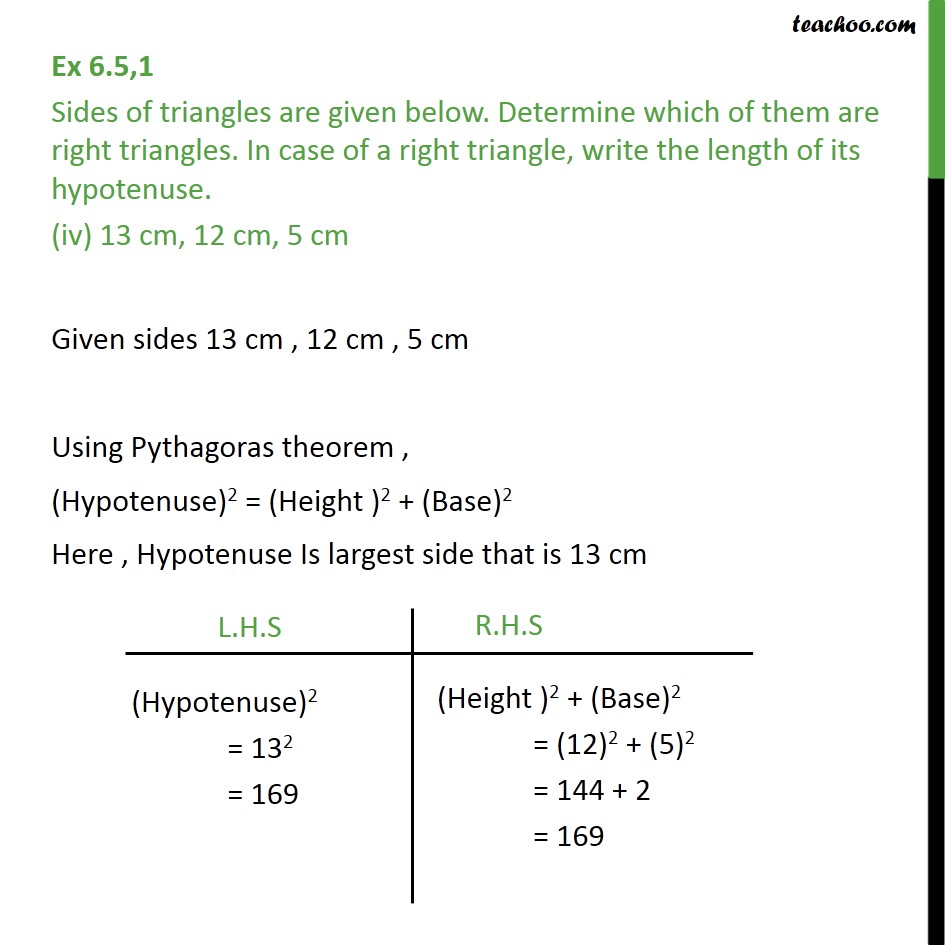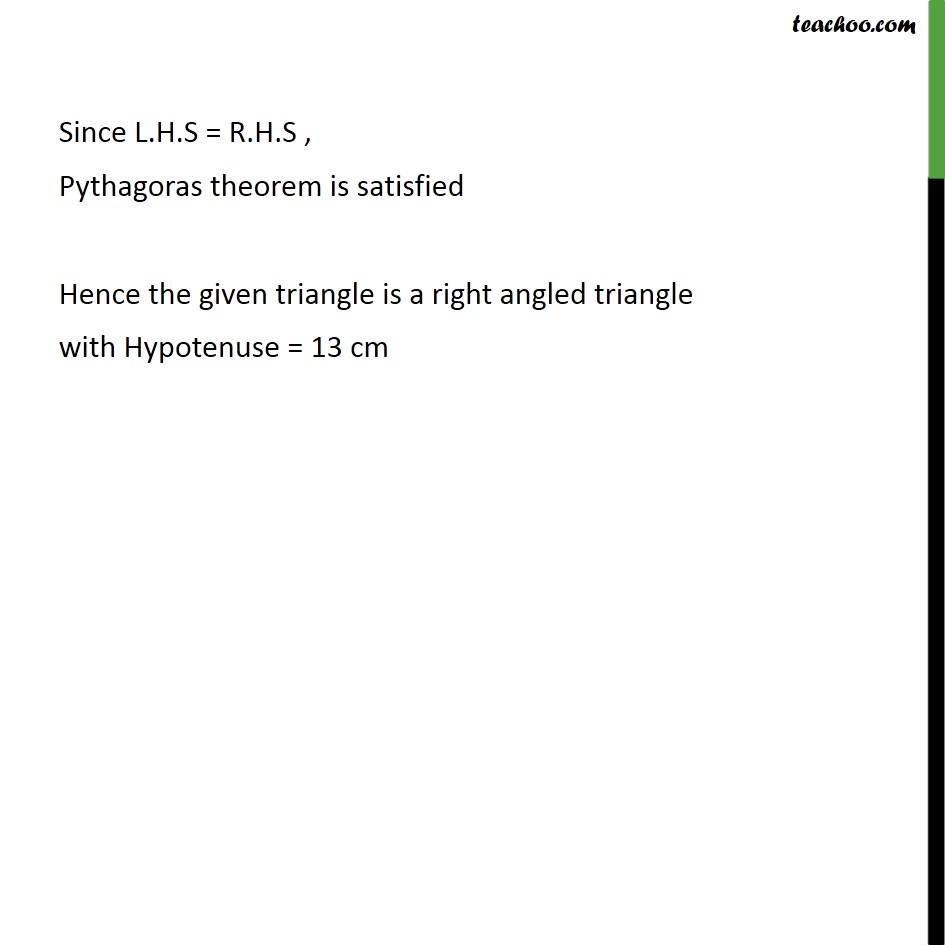Introducing your new favourite teacher - Teachoo Black, at only ₹83 per month

### Transcript

Ex 6.5,1 Sides of triangles are given below. Determine which of them are right triangles. In case of a right triangle, write the length of its hypotenuse. (i) 7 cm, 24 cm, 25 cm Given sides 7 cm , 24 cm , 25 cm Using Pythagoras theorem , (Hypotenuse)2 = (Height )2 + (Base)2 Here , Hypotenuse Is largest side that is 25 cm Since L.H.S = R.H.S , Pythagoras theorem is satisfied Hence the given triangle is a right angled triangle with Hypotenuse = 25 cm Ex 6.5,1 Sides of triangles are given below. Determine which of them are right triangles. In case of a right triangle, write the length of its hypotenuse. (ii) 3 cm, 8 cm, 6 cm Given sides 3 cm , 8 cm , 6 cm Using Pythagoras theorem , (Hypotenuse)2 = (Height )2 + (Base)2 Here , Hypotenuse Is largest side that is 8 cm Since L.H.S ≠ R.H.S , Pythagoras theorem is not satisfied Hence the given triangle is not a right angled triangle Ex 6.5,1 Sides of triangles are given below. Determine which of them are right triangles. In case of a right triangle, write the length of its hypotenuse. (iii) 50 cm, 80 cm, 100 cm Given sides 50 cm , 80 cm , 100 cm Using Pythagoras theorem , (Hypotenuse)2 = (Height )2 + (Base)2 Here , Hypotenuse Is largest side that is 100 cm Since L.H.S ≠ R.H.S , Pythagoras theorem is not satisfied Hence the given triangle is not a right angled triangle Ex 6.5,1 Sides of triangles are given below. Determine which of them are right triangles. In case of a right triangle, write the length of its hypotenuse. (iv) 13 cm, 12 cm, 5 cm Given sides 13 cm , 12 cm , 5 cm Using Pythagoras theorem , (Hypotenuse)2 = (Height )2 + (Base)2 Here , Hypotenuse Is largest side that is 13 cm Since L.H.S = R.H.S , Pythagoras theorem is satisfied Hence the given triangle is a right angled triangle with Hypotenuse = 13 cm